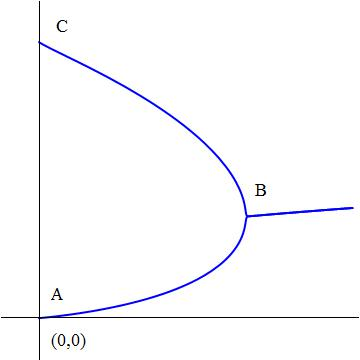# Numerical Integration of Exponential Tetration

Calculus Level 5

An exponential tetration is where $x$ is exponentiated by itself $n$ times.For example,

$_{ }^{ 4 }{ x }={ x }^{ x }^{ x }^x } }$

As $n$ approaches $\infty$, then for some $0, the function bifurcates at point $B$, splitting into the upper branch where $n$ is even and the lower branch where $n$ is odd, even though in both cases, $n$ approaches $\infty$. Point $A$ is the origin $(0,0)$ and point $C$ is $(0,1).$ Let $T$ be the area of $ABC$ as defined by the upper and lower branches, and the line $x=0.$ What is the floor value $\left\lfloor 10000T \right\rfloor?$

Note: Use of a computer and software is expected for numerical integration. The figure above is not drawn to scale.

×